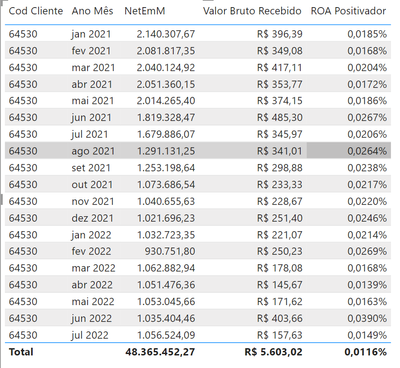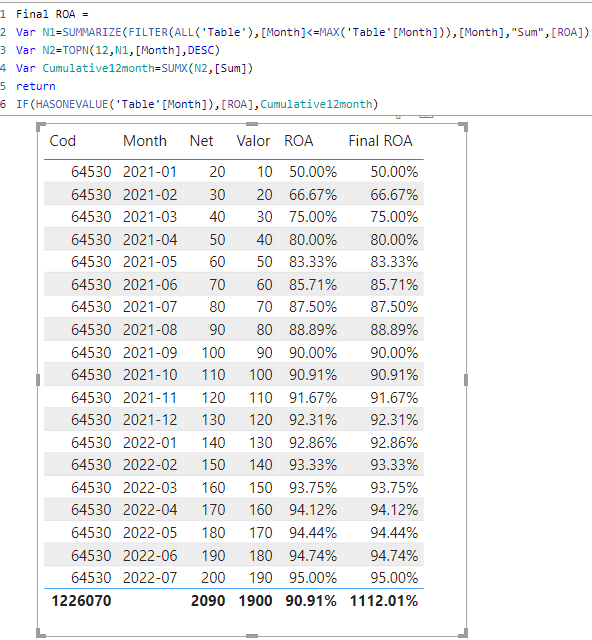cancel
Showing results for
Did you mean:Frequent Visitor

## How to calculate subtotal sum of a measure?

Hi guys,

I have a measure (ROA) which is calculated by dividing revenue with clients NET position. I need a way to display this measure by summing the last 12 months from its result.However as you can see on the total bottom right it is aggregating it by division from the total.

DIVIDE(
SUM('Historico Receita'[Valor Bruto Recebido]),
)

Any ideas on how to solve this? Thanks in advance.

1 ACCEPTED SOLUTIONCommunity Support

Do you want the total ROA to show the sum of divisions for the last 12 months? You can try the following methods.

Sample data:Measure:

``ROA = DIVIDE(SUM('Table'[Valor]),SUM('Table'[Net]))``
``````Final ROA =
Var N1=SUMMARIZE(FILTER(ALL('Table'),[Month]<=MAX('Table'[Month])),[Month],"Sum",[ROA])
Var N2=TOPN(12,N1,[Month],DESC)
Var Cumulative12month=SUMX(N2,[Sum])
return
IF(HASONEVALUE('Table'[Month]),[ROA],Cumulative12month)``````Is this the result you expect?

Best Regards,

Community Support Team _Charlotte

If this post helps, then please consider Accept it as the solution to help the other members find it more quickly.

2 REPLIES 2Community Support

Do you want the total ROA to show the sum of divisions for the last 12 months? You can try the following methods.

Sample data:Measure:

``ROA = DIVIDE(SUM('Table'[Valor]),SUM('Table'[Net]))``
``````Final ROA =
Var N1=SUMMARIZE(FILTER(ALL('Table'),[Month]<=MAX('Table'[Month])),[Month],"Sum",[ROA])
Var N2=TOPN(12,N1,[Month],DESC)
Var Cumulative12month=SUMX(N2,[Sum])
return
IF(HASONEVALUE('Table'[Month]),[ROA],Cumulative12month)``````Is this the result you expect?

Best Regards,

Community Support Team _Charlotte

If this post helps, then please consider Accept it as the solution to help the other members find it more quickly.Super User

@diogodasilva This looks like a measure totals problem. Very common. See my post about it here: https://community.powerbi.com/t5/DAX-Commands-and-Tips/Dealing-with-Measure-Totals/td-p/63376

Also, this Quick Measure, Measure Totals, The Final Word should get you what you need:
https://community.powerbi.com/t5/Quick-Measures-Gallery/Measure-Totals-The-Final-Word/m-p/547907

Become an expert!: Enterprise DNA
External Tools: MSHGQM
Latest book!:
Mastering Power BI 2nd EditionDAX is easy, CALCULATE makes DAX hard...